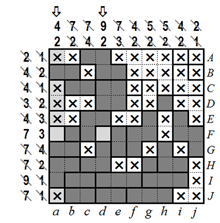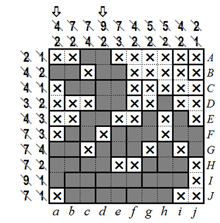﻿ Cross-a-Pix решение
 ВходРегистрация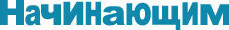Поддержка Adobe Flash Player

В связи с окончанием поддержки Flash Player всеми браузерами к концу 2020 года, мы переводим наши игры на технологию HTML5. Переход будет завершен осенью 2019 года. Пока же, если вы сталкиваетесь с проблемами активации Flash Player, читайте здесь

Cross-a-Pix решение

Cross-a-Pix puzzles consist of a grid divided into various regions, where each region is either filled or left empty according to the rules. When the puzzle is completed, a hidden pixilated picture is revealed. Cross-a-Pix puzzles come in two variants, SingleClue and DualClue. SingleClue Cross-a-Pix use one clue in each row and column to show how many squares are painted while DualClue Cross-a-Pix use two clues in each row and column to show how many squares are painted and into how many blocks they are divided.

SingleClue Cross-a-Pix step by step solution

SingleClue Cross-a-Pix puzzles are solved by looking at a row or a column, one at a time, analyzing the clue and the regions, and working out which blocks must be painted and which must remain empty. It is just as important marking empty regions with X or a dot as it is painting the regions which must be filled. The best way to learn how to solve SingleClue Cross-a-Pix puzzles is to see a puzzle solved step by step from beginning to end.

Step 1

Solving SingleClue Cross-a-Pix starts by looking for squares which are obviously filled or empty and then painting (or marking with an X or a dot) all remaining squares belonging to the respective region. Looking at column b below we see that whichever way we paint 8 out of the 10 squares, at least 3 of them will be inside the block of 5. Since according to Cross-a-Pix rules all squares in a region must be the same, all 5 squares in this block are painted. Now let’s look at column d, in which 9 out of the 10 squares must be painted. In this case 3 blocks consisting of 2 squares each, as well as 2 additional squares belonging to the region of the block in the center, are painted as shown below on the right.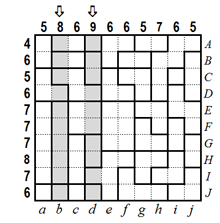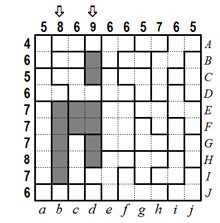Step 2

In row E below, 3 of the 7 squares have been filled in the previous step so 4 more squares must be painted. However, this is only possible if the leftmost square in this row is empty… otherwise the number of added squares will always be odd. We therefore mark the leftmost square of row E with an X to indicate it is empty. Also, since according to Cross-a-Pix rules all squares in a region must be the same, we can mark the remaining 4 squares of that region with an X to indicate they are empty as well.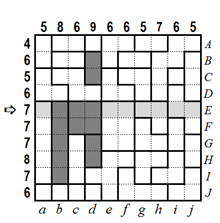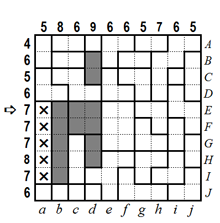Step 3

The next step is column a, in which 5 squares are now marked as empty. This means all remaining 5 squares in that column, as well as the squares belonging to their regions, can be painted. Although not immediately obvious, there is only one way to paint 6 out of the 10 squares in column e. The 6 squares are painted, as well as the squares belonging to their regions. Similarly, the remaining 4 squares in column e, as well as the squares belonging to their regions, are marked with X to indicate they are empty. Finally, the clues of columns a and e are checked off. It is recommended to check off clues of completed rows and columns to indicate they are no longer needed and to avoid confusions later on.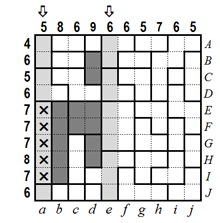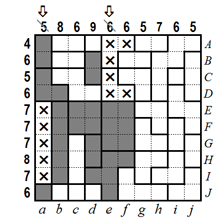Step 4

In row A below, 1 of the 4 squares has been filled in the previous steps so 3 more squares must be painted. However, this is only possible if the square in column d is filled… otherwise the number of added squares will always be even. Using the same logic, we mark the rightmost square of row B with an X, as well as the remaining two squares of its region, to indicate they are empty. Lastly, of the 5 remaining empty squares in row H, 4 must be filled. This means that the block of 2, as well as the square belonging to its region, must be painted.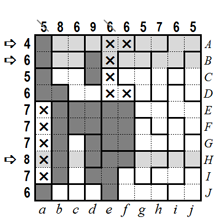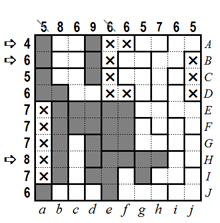Step 5

In column j below, 5 squares must be painted. However, this is only possible if the topmost square is filled. We therefore paint the topmost square of column j as well as the squares belonging to its region.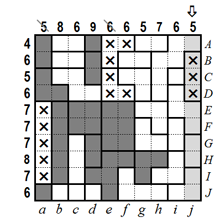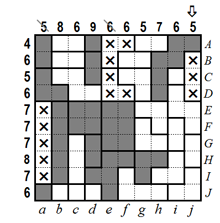Step 6

Row A now has 4 painted squares, so we can mark the remaining squares with an X, as well as the corresponding squares belonging to their regions, to indicate they are empty. In addition, we mark the second square from the right in row C with an X because otherwise it would not be possible to have 5 painted squares in this row.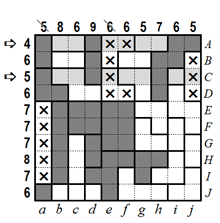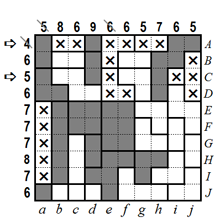Step 7

Using same logic as above, the bottom square in column i must be empty. We mark this square with an X, as well as the 2 remaining squares belonging to its region, to indicate they are empty.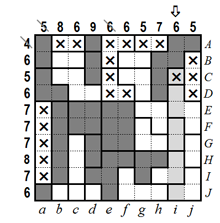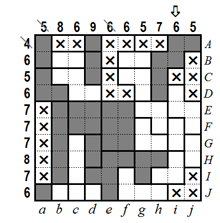Step 8

Using same logic as in the previous steps, we can now paint additional squares in rows D and J, as well as the squares belonging to their respective regions.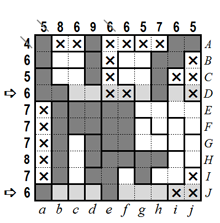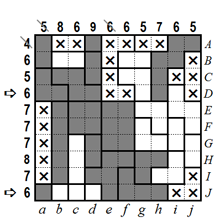Step 9

Columns c, f and j can now be finished and their clues checked off to indicate they are completed.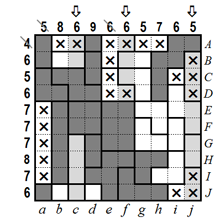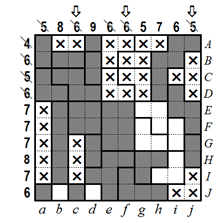Step 10

Using the same techniques as before, we can now complete the puzzle. The SingleClue Cross-a-Pix puzzle is solved, revealing the picture of a cat.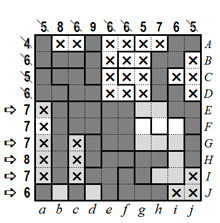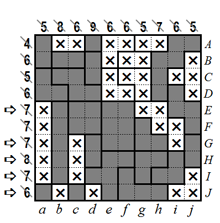DualClue Cross-a-Pix step by step solution

DualClue Cross-a-Pix puzzles are solved by looking at a row or a column, one at a time, analyzing the two clues showing how many squares should be painted and into how many blocks they should be divided, and working out which blocks must be painted and which must remain empty. It is just as important marking empty regions with X or a dot as it is painting the regions which must be filled. The best way to learn how to solve DualClue Cross-a-Pix puzzles is to see a puzzle solved step by step from beginning to end.

Step 1

Solving DualClue Cross-a-Pix starts by looking for squares which are obviously filled or empty and then painting (or marking with an X or a dot) all remaining squares belonging to the respective region. In row A below we need to paint 1 block of 2 squares. However, this is not possible in the block of 4 squares since according to the rules of Cross-a-Pix, all squares in a region must be the same. We therefore mark the 4 squares in row A with an X, as well as the remaining 4 squares belonging to that region, to indicate they are all empty. In row I, 9 out of the 10 squares must be painted. This means either the square on the left of this row or the square on the right of this row are empty. We can therefore paint the 3 blocks in the middle of this row as shown below.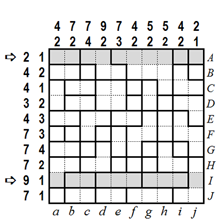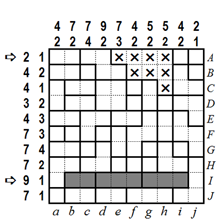Step 2

In column d, 9 squares must be painted in 2 blocks. Even if we ignore the requirement to have 2 blocks, it is immediately obvious that there can be no empty blocks longer than 1. We can therefore paint the 3 blocks of 2 squares in this column as shown below. In column j we need to paint 1 block of 2 squares. However, this is not possible in the bottom block which is 3 squares long. We therefore mark the 3 squares in column j with an X, as well as the remaining square belonging to that region, to indicate they are empty.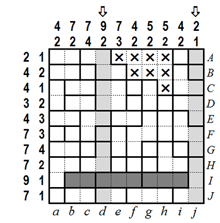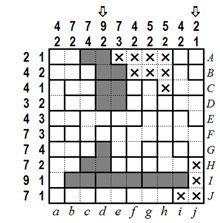Step 3

The next step is row A again, in which 2 squares are now painted from the previous step. This means the 4 remaining empty squares can be marked with an X and the clues checked off to indicate this row is completed. Row I is also almost done. We can paint the remaining empty square, as well as the 2 squares belonging to that region, and check off the clues to indicate this row is completed.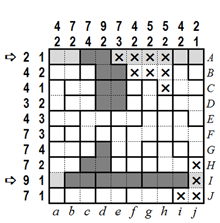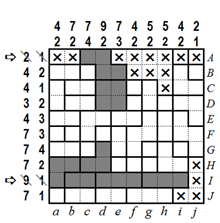Step 4

In row C below there is 1 block of 4 squares, of which 2 squares have already been painted in the previous steps. This means the square on the extreme left and the two squares on the extreme right of this row must be empty. We can therefore mark these 3 squares with an X, as well as the remaining squares belonging to their regions, to indicate they are empty. Looking at row J we see there is only one way to fill 7 squares according to the rules. We paint the 7 squares, mark the leftmost square with an X, and check off the clues to indicate this row is completed.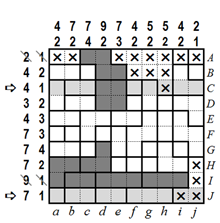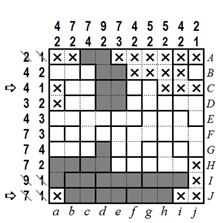Step 5

Looking at column e we see there is only one way to paint the missing 2 squares so there are 7 squares in 3 blocks. We can therefore finish this column and check off the clues to indicate it is completed. In column h, 2 of the 5 squares have been filled in the previous steps so 3 more squares must be painted. However, this is only possible if the topmost empty square is painted… otherwise the number of added squares will always be even.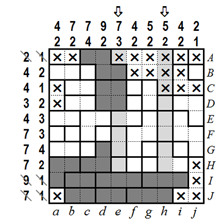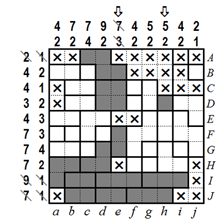Step 6

The next step is row D, in which 3 squares in 2 blocks have been painted in the previous steps. We can now complete this row by marking the 6 remaining empty squares with an X, as well as the squares belonging to their respective regions. In row H we already have 4 painted squares which means that 3 additional squares must be painted. However, any way we try to paint 3 squares, the block of 2 on the right must be filled as shown below.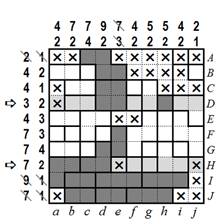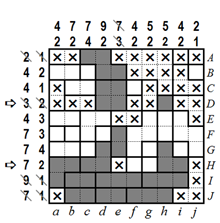Step 7

We can now make progress with columns g, h and j, using the same logic as the previous steps.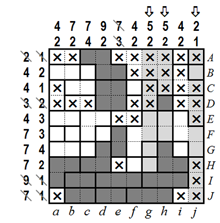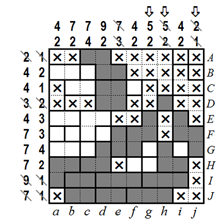Step 8

Similarly, rows B, C and H can now be completed and their clues checked off.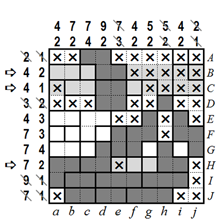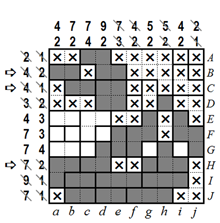Step 9

In column b there are still 2 squares to be painted. However, there is only one way to do it if we want to have 7 painted squares in 2 blocks. We therefore paint the 2 bottom squares and the squares belonging to their regions, and mark the remaining top square with an X. Next, we can complete the last empty square in column g and the last empty square in column i and check off the respective clues.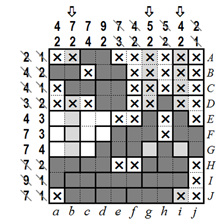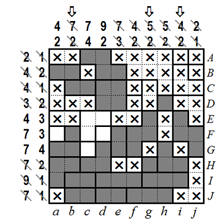Step 10

Rows E and G can now be completed and their clues checked off.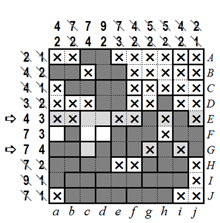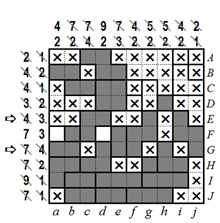Step 11

And lastly, columns a and d can now be completed. The DualClue Cross-a-Pix puzzle is now solved, revealing the picture of a swan.